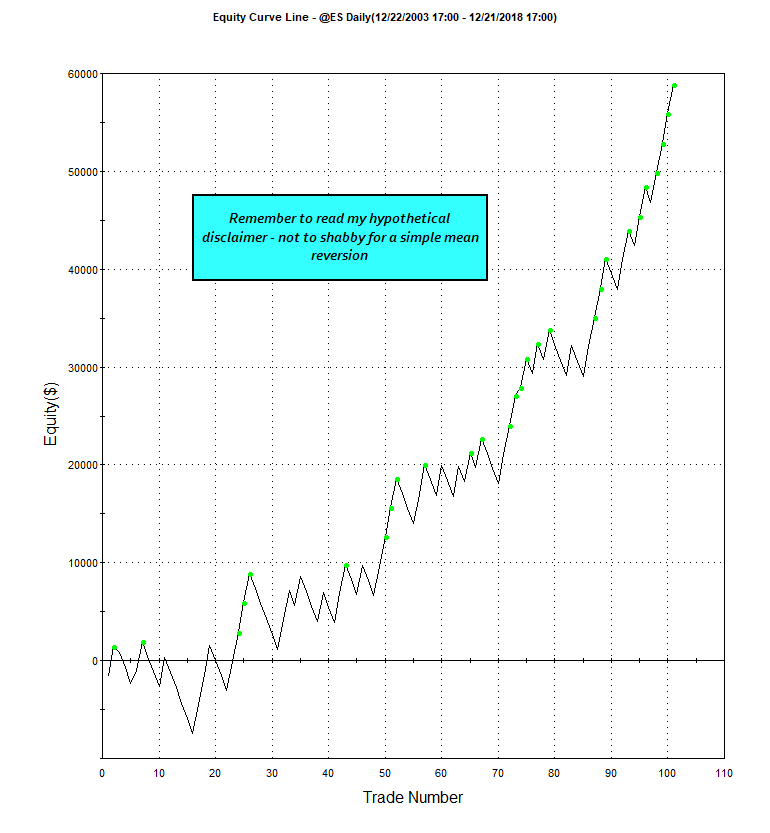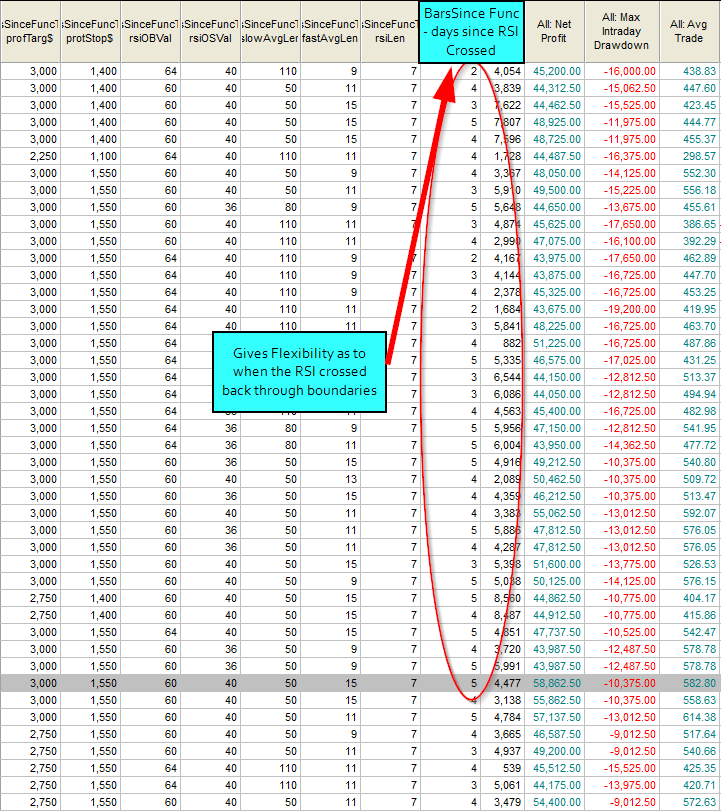# George’s EasyLanguage BarsSince Function – How Many Bars Since?

Have you ever wondered how many bars have transpired since a certain condition was met?  Some platforms provide this capability:

If ExitFlag and (c crosses above average within 3 bars) then

TradeStation provides the MRO (Most Recent Occurrence) function that provides a very similar capability.  The only problem with this function is that it returns a -1 if the criteria are not met within the user provided lookback window.  If you say:

myBarsSinceCond = MRO(c crosses average(c,200),20,1) < 3

And c hasn’t crossed the 200-day moving average within the past twenty days the condition is still set to true because the function returns a -1.

I have created a function named BarsSince and you can set the false value to any value you wish.  In the aforementioned example, you would want the function to return a large number so the function would provide the correct solution.  Here’s how I did it:

``````inputs:
Test( truefalseseries ),
Length( numericsimple ),
Instance( numericsimple ) , { 0 < Instance <= Length}

value1 = RecentOcc( Test, Length, Instance, 1 ) ;
If value1 = -1 then
Else

And here’s a strategy that uses the function:

``````inputs: profTarg\$(2000),protStop\$(1000),
rsiOBVal(60),rsiOSVal(40),slowAvgLen(100),

Value1 = BarsSince(rsi(c,rsiLen) crosses above rsiOSVal,rsiLen,1,999);
Value2 = BarsSince(rsi(c,rsiLen) crosses below rsiOBVal,rsiLen,1,999);

If c > average(c, slowAvgLen) and c < average(c,fastAvgLen) and Value1 <barsSinceMax then buy next bar at open;

If c < average(c, slowAvgLen) and c > average(c,fastAvgLen) and Value2 <barsSinceMax then sellshort next bar at open;

setStopLoss(protStop\$);
setProfitTarget(profTarg\$)``````

The function requires four arguments:

1. The condition that is being tested [e.g.  rsi > crosses above 30]
2. The lookback window [rsiLen – 14 bars in this case]
3. Which occurrence [1 – most recent; 2- next most recent; etc…]
4. False return value [999 in this case; if condition is not met in time]

## A Simple Mean Reversion Using the Function:

Here are the results of this simple system utilizing the function.### Optimization Results:

I came up with this curve through a Genetic Optimization:The BarsSince function adds flexibility or fuzziness when you want to test a condition but want to allow it to have a day (bar) or two tolerance.  In a more in-depth analysis, the best results very rarely occurred on the day the RSI crossed a boundary.   Email me with questions of course.